BenQ Knowledge Center

## Definition of Gamma

Gamma can be described as how smoothly black transits to white on a digital display. It is often associated with a number like 2.2 or 2.4, and this number represents the shape of the curve from black to white, or from white to black. Some typical gamma curves are shown in Figure 1, and the equation of obtaining the gamma curve value is shown in equation 1.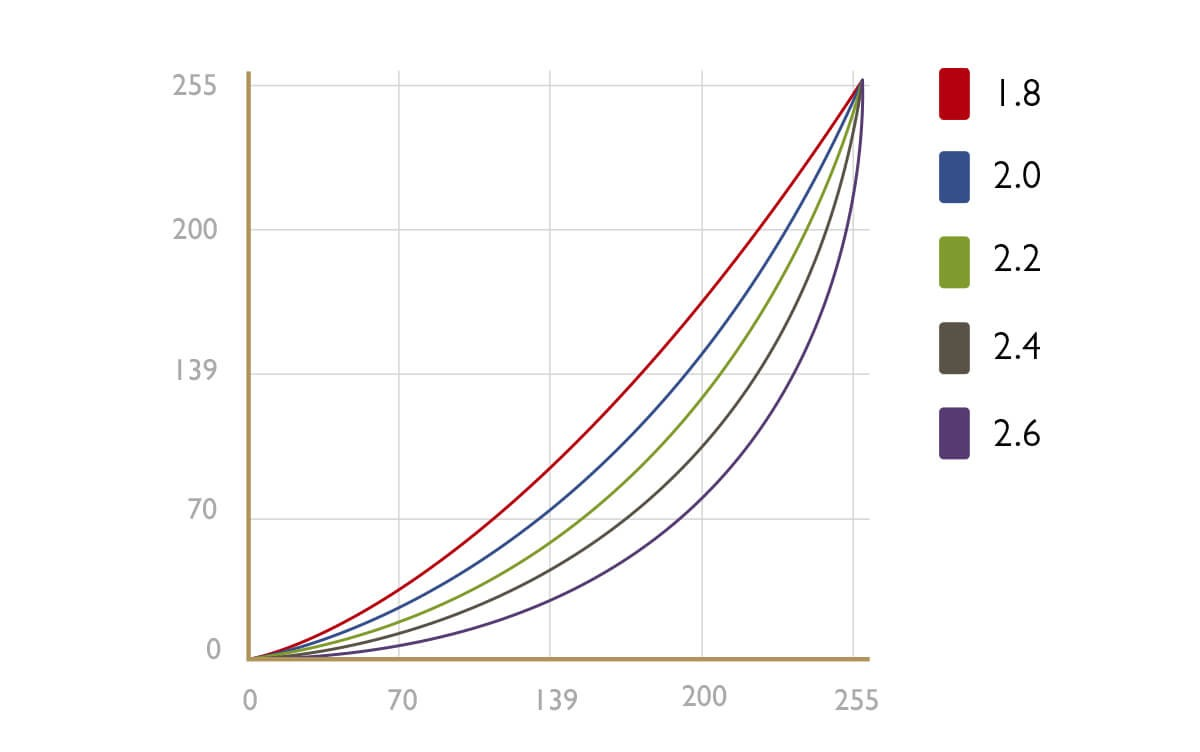Figure 1: Different Gamma Values and Its Associated Curves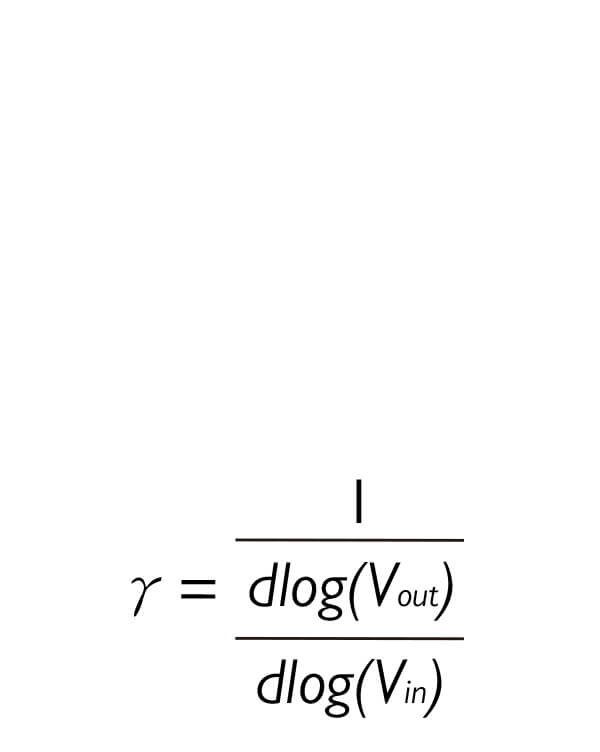Equation 1:  Gamma Curve Value

## Why Gamma 2.2 is a standard?

Many of you may wonder why a standard gamma curve is defined as gamma 2.2. The main reason that a power-law relationship exists between the output luminance and the input voltage or digital value is because the visual system does not operate in a linear way. Let’s take a look at Figure 2. At the bottom row, linear intensity represents the intensity increase from black to white in a linear fashion. At the top row, visual encoding represents the intensity increase from black to white in a power-law fashion. Please note that between 0.0 and 0.1, there is a big visual gap in linear intensity whereas it is much less apparent between 0.0 and 0.1 in visual encoding. And from 0.9 to 1.0 in linear intensity, the difference is not perceivable, whereas it is perceivable in visual encoding. Looking at the overall grays in the visual encoding row, the perceived differences between each gray patch are almost identical. This phenomenon was also found in Ebner and Fairchild’s study in 1998, where they used an exponent of 0.43 to convert linear intensity into lightness for neutrals to provide an optimal perceptual encoding of grays. And the exponent of 0.43 is approximately 2.33 and is quite close to gamma 2.2. Hence, the gamma value of 2.2 has become the golden standard of digital displays for proper calibration.Figure 2: Visual Differences in Visual Encoding and Linear Intensity

## Gamma Curve and Visual Perception

So how does the gamma curve impact overall image quality or perception? Gamma 2.2 delivers a balanced or ‘neutral’ tone between highlights and shadows, and you can distinguish the grays easily in between. Gamma 1.0 is an interesting curve to look at. This is a 45-degree straight line relationship between the input signal and output luminance. This is also a ‘by-pass’ scenario where no processing is done on the display end. So you will see a very bright and ‘flat’ image, where almost no contrast is present, such as in Figure 3.Figure 3: Images of Gamma 1.0 (on the left) compared to Gamma 2.2 (on the right)

Gamma 1.8 was very popular due to Mac OS. The gamma 1.8 curve produces slightly brighter images than gamma 2.2 so sometimes it is more preferred in some cases. However, since Mac OS X 10.6, gamma 2.2 has become the standard gamma curve for Mac OS as well. An example of gamma 1.8 versus gamma 2.2 is illustrated in Figure 4.Figure 4Images of Gamma 1.8 (on the left) compared to Gamma 2.2 (on the right)

Gamma 2.4 is widely used in the movie and TV industries due to the Rec. 709 standard. The slightly enhanced contrast brings out the saturation of colors and stimulates viewer perception and preference. However, the overall brightness of images may be lowered. An example of gamma 2.4 versus gamma 2.2 is illustrated in Figure 5.Figure 5: Images of Gamma 2.4 (on the left) compared to Gamma 2.2 (on the right)

Gamma 2.6 has started to gain in popularity because of the latest DCI-P3 standard. DCI-P3 is endorsed by many newer digital cinema theaters. With a gamma 2.6 curve, images definitely look darker but very saturated. And as this is the effect required by directors, DCI-P3 needs a gamma 2.6 curve. An example of gamma 2.6 versus gamma 2.2 is illustrated in Figure 6.Figure 6: Images of Gamma 2.6 (on the left) compared to Gamma 2.2 (on the right)

Nevertheless, what will happen if a gamma curve is not smooth? If a gamma curve is not smooth; that means the transition from black to white is not strictly incremental, as shown in Figure 7. Then the visual differences in grayscale will look not perceptually different, and also with coloration (not neutral gray). And when this phenomenon applies to a grayscale image, the image will lose detail and result in a different image than it is supposed to be.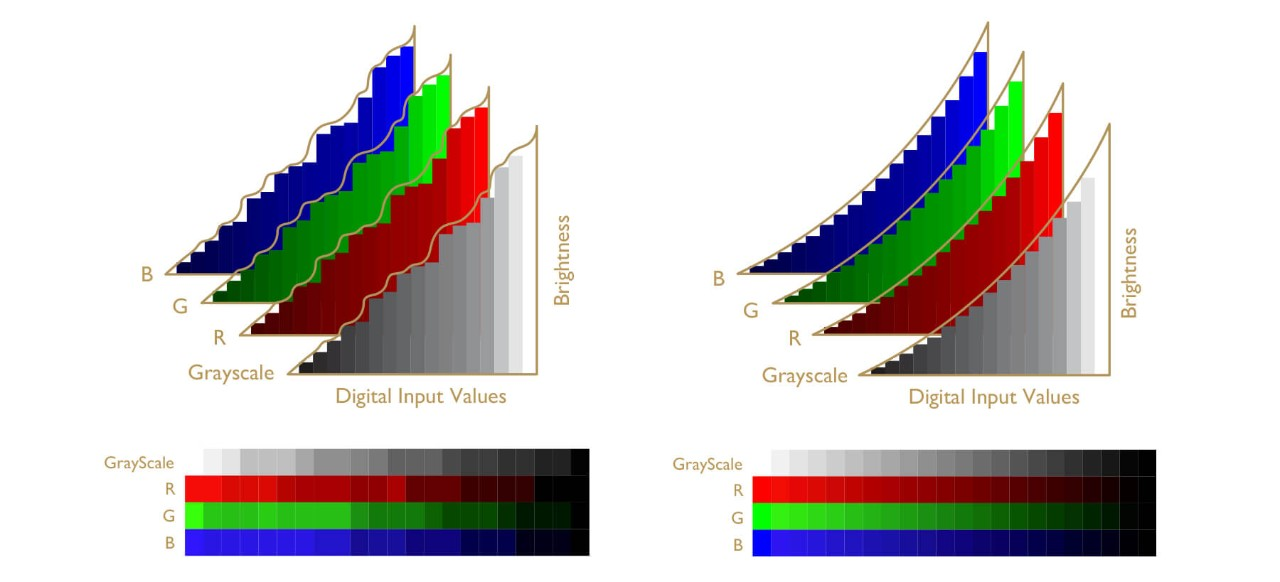Figure 7: a gamma curve that isn't smooth can result in artifacts in perceptual grayscale and RGB ramps (on the left). No artifact present in perceptual grayscale and RGB ramps when the gamma curve is smooth (on the right).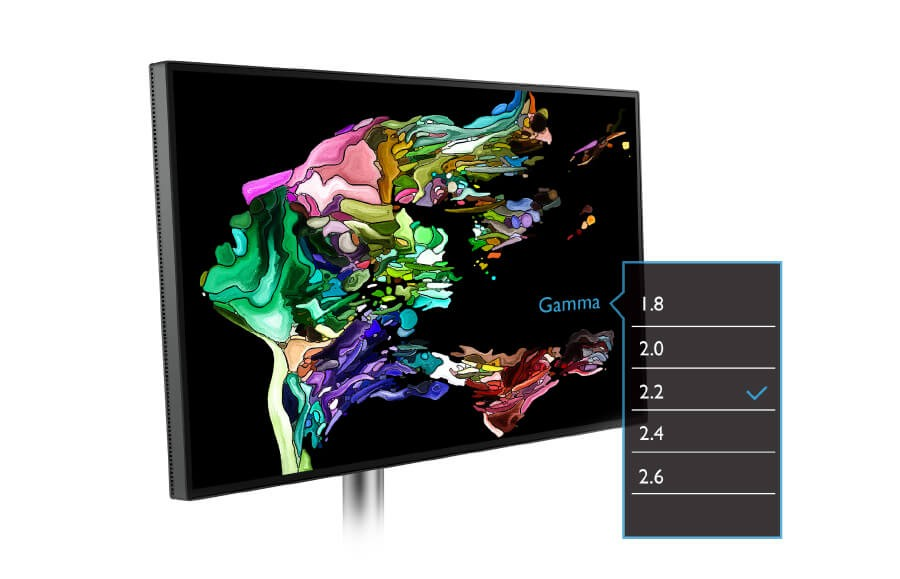Figure 8: Select preferred gamma via monitor OSD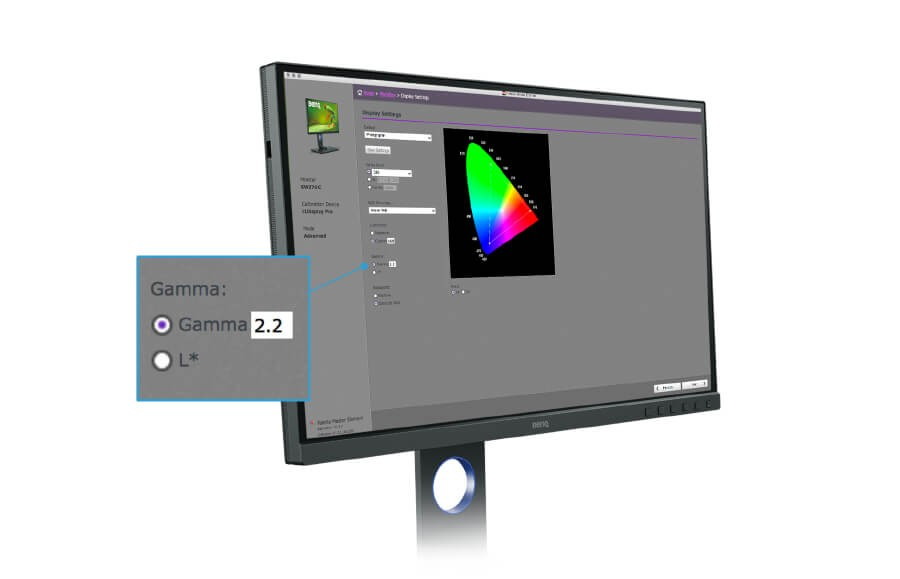Figure 9: Select preferred gamma with

BenQ Palette Master Element software

Since we now have some basic ideas of how gamma curves impact image quality and how important it is to have a good gamma curve, how do we adjust the gamma curve? Usually there are two ways of doing that. The first way is straightforward, you can just look for the OSD on you monitor. Usually on a professional color management monitor, such as BenQ SW series monitors or PD series monitors, there is a ‘Custom Mode’ in the ‘Color Mode’ option. In ‘Custom Mode’, you are can select different settings such as color gamut or gamma curve. Under the ‘Gamma’ option, you can select factory calibrated gamma curves, ranging from 1.6 to 2.6 with 0.2 intervals.

Another way of adjusting the gamma curve on SW series monitors is to utilize BenQ proprietary calibration software, Palette Master Element. Connecting with an external calibrator, such as X-rite i1 Display Pro, i1 Pro 2, or a Datacolor Spyder 5, you can simply select the gamma curve you would like in Advanced Mode. Following the instructions on the screen, after completing the calibration, you can be sure your gamma curve is ready for you to use in Calibration 1, 2, or 3. It is as simple as that. This is the preferred method to adjust your gamma curve if you have one of our SW series monitors.

Summary

We talked about the definition of gamma curve in the early paragraphs of this article, and its important link to visual response. We also looked at how gamma curve can affect image quality and perception, and some typical gamma values and their applications. Lastly, we learned the importance of maintaining a smooth gamma curve and how to select different gamma curves on BenQ SW/PD monitors. With Palette Master Element software and an external calibrator, you can also calibrate your gamma curve on SW monitors.

TOP